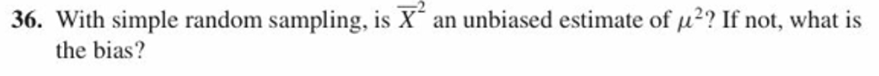# 36. With simple random sampling, is X an unbiased estimate of u2 If not, what isthe bias?

Question
65 viewshelp_outlineImage Transcriptionclose36. With simple random sampling, is X an unbiased estimate of u2 If not, what is the bias? fullscreen
check_circle

Step 1

Check whether sample mean square is an unbiased estimator of population mean square or not.

Unbiased estimator:

Any statistic is said to be an unbiased estimator of the specified parameter, if the expected value of the sampling distribution of that statistic is equal to the parameter being estimated. In other words it can be said that, an unbiased estimator is an estimator with “0” bias.

The sample mean is an unbiased estimator of population mean.

That is, E(X-bar) = μ.

Consider the  X = (X1, X2,…, Xn) be the sample drawn from a population of mean μ.

The sample mean be denoted by X-bar.

Here, the claim is that whether the sample mean square is an unbiased estimator of population mean square or not.

The sample mean square is proved to be not an unbiased estimator of population mean square from the calculation given below:

Step 2

Obtain the bias in the estimator sample mean square:

Bias:

Bias of an estimator is the difference between estimator’s expected value and the true value of the parameter that is bein...

### Want to see the full answer?

See Solution

#### Want to see this answer and more?

Solutions are written by subject experts who are available 24/7. Questions are typically answered within 1 hour.*

See Solution
*Response times may vary by subject and question.
Tagged in

### Other